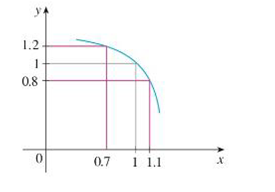Chapter 2.4, Problem 1E### Single Variable Calculus: Early Tr...

8th Edition
James Stewart
ISBN: 9781305270343

#### Solutions

Chapter
Section### Single Variable Calculus: Early Tr...

8th Edition
James Stewart
ISBN: 9781305270343
Textbook Problem

# Use the given graph of f to find a number δ such thatif |x – 1| < δ then |f(x) – 1| < 0.2To determine

To find: The value of a number δ by using the graph of f.

Explanation

Given:

If |x1|<δ, then |f(x)1|<0.2.

Definition used:

If for every number ε>0 there is a number δ>0 such that if 0<|xa|<δ, then |f(x)L|<ε.

Absolute value inequality: If |x|<a,a>0, then a<x<a.

Calculation:

Consider |f(x)1|<0.2.

By absolute value definition, 0.2<f(x)1<0.2.

Simplify the inequality by adding 1 on each side,

10.2<1+f(x)1<1+0

### Still sussing out bartleby?

Check out a sample textbook solution.

See a sample solution

#### The Solution to Your Study Problems

Bartleby provides explanations to thousands of textbook problems written by our experts, many with advanced degrees!

Get Started

#### A sample of n = 9 scores has X = 108. What is the sample mean?

Essentials of Statistics for The Behavioral Sciences (MindTap Course List)

#### 13. If , find n.

Mathematical Applications for the Management, Life, and Social Sciences

#### Describe how replication protects against fraud being committed in research.

Research Methods for the Behavioral Sciences (MindTap Course List)